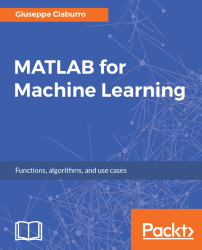•#### MATLAB for Machine Learning#### Overview of this book

MATLAB is the language of choice for many researchers and mathematics experts for machine learning. This book will help you build a foundation in machine learning using MATLAB for beginners. You’ll start by getting your system ready with t he MATLAB environment for machine learning and you’ll see how to easily interact with the Matlab workspace. We’ll then move on to data cleansing, mining and analyzing various data types in machine learning and you’ll see how to display data values on a plot. Next, you’ll get to know about the different types of regression techniques and how to apply them to your data using the MATLAB functions. You’ll understand the basic concepts of neural networks and perform data fitting, pattern recognition, and clustering analysis. Finally, you’ll explore feature selection and extraction techniques for dimensionality reduction for performance improvement. At the end of the book, you will learn to put it all together into real-world cases covering major machine learning algorithms and be comfortable in performing machine learning with MATLAB.
Title PageCreditsForewordwww.PacktPub.comCustomer FeedbackPrefaceFree Chapter
Getting Started with MATLAB Machine LearningImporting and Organizing Data in MATLABFrom Data to Knowledge DiscoveryFinding Relationships between Variables - Regression TechniquesPattern Recognition through Classification AlgorithmsIdentifying Groups of Data Using Clustering MethodsSimulation of Human Thinking - Artificial Neural NetworksImproving the Performance of the Machine Learning Model - Dimensionality ReductionMachine Learning in Practice## Summary

In this chapter, we learned how to simulate typical human brain activities through the ANN. We understood the basic concept of ANN. We saw how to build a simple neural network architecture. We explored topics such as input, hidden, and output layers; weights of connections; and the activation function.

We learned how to choose the number of hidden layers, the number of nodes within each layer, and the network training algorithm. Then, we took a tour into of the Neural Network Toolbox (which provides algorithms), pre-trained models, and apps to create, train, visualize, and simulate shallow, as well as deep, neural networks. We checked out the neural network getting started GUI, the starting point for our neural network fitting, pattern recognition, clustering, and time series analysis.

Finally, we focused on fitting data with a neural network. We saw how to use the Neural Fitting app (`nftool`). Then, we ran a script analysis to learn how to use neural network functions from the command...